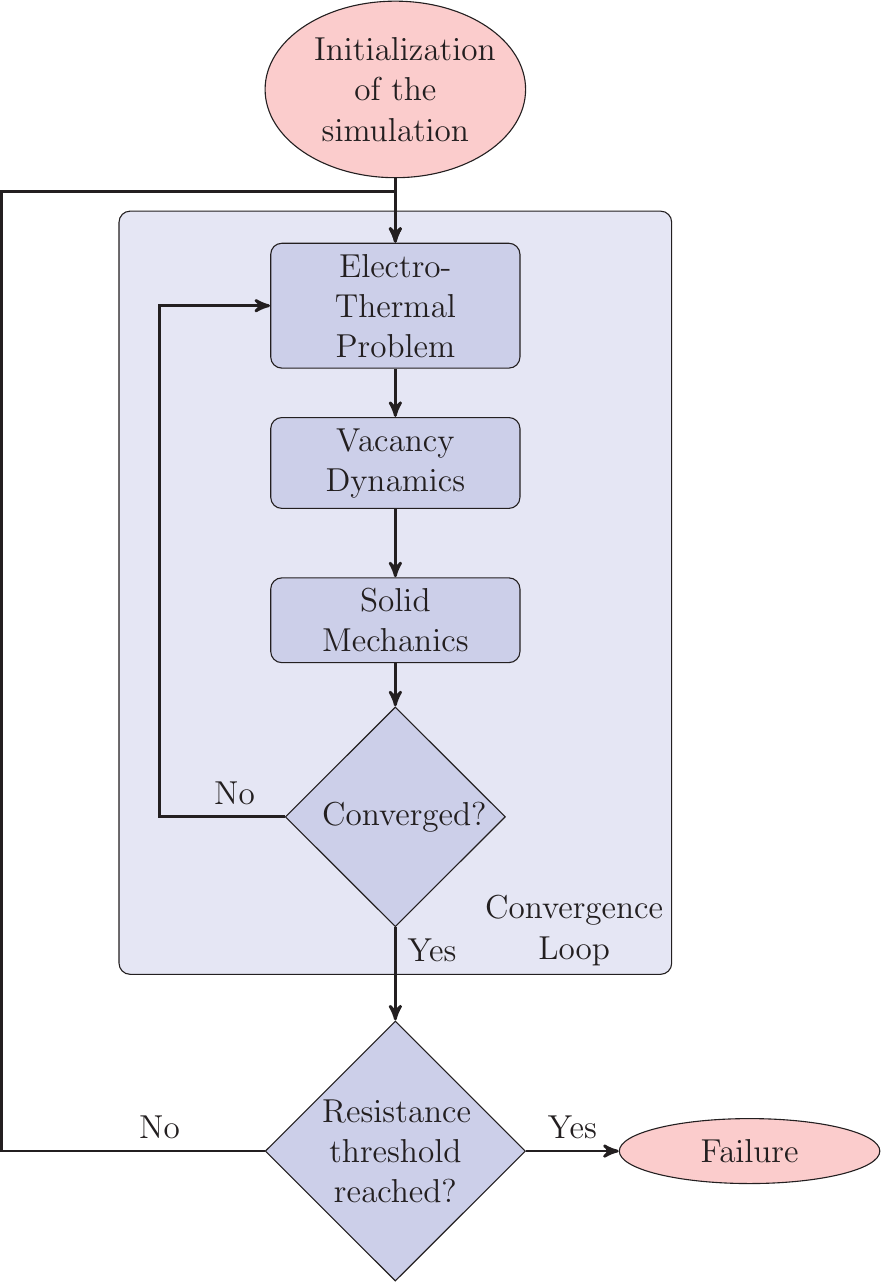Previous: 4.2 Implementation in FEDOS    Top: 4 Numerical Implementation    Next: 5 Results and Discussion

#### 4.3  Implementation in COMSOL

COMSOL  is a commercial program widely used in industry and research for the simulation of FEM based problems. It offers a complete solution for various physics and engineering applications starting from the geometry generation over the modeling to the simulation and evaluation with various plotting tools and data exporting capabilities. In addition it offers a material data base from where material parameters can be imported.

##### 4.3.1  Electro-Mechanical Model

The electro-mechanical model is a preconfigured model including the electric potentialwherestands for the electric potential,for the current density,for a user predefined current density,for the free charge distribution andfor the conductivity. The Joule heating modeling is formulated by the equation systemwherestand for the thermal energy dissipated per time,for the thermal expansion coefficient under constant pressure,for the volumetric mass density,for the thermal conductivity, andfor the velocity field. The mechanical stress modeling is implementedFigure 4.5.:  Flowchart of the EM simulation in FEDOS.

for small deformations bywherestands for the body force density,for the reference temperature of the thermal expansion, andfor the strain originating from the thermal expansion. This model has the ability to include strains byor stresses byfrom another model and is used to includes the strain arising from the vacancy flux and vacancy generation/annihilation in the EM model.

##### 4.3.2  PDE as Electromigration Model

Since not for all physical problems models are included in COMSOL, it provides the “Coefficient Form PDE”, which allows to express a huge set of technical relevant linear and non-linear PDEs. This general PDE has the following form:EM is represented by two differential equations, one for the vacancy flux and one for the strain due to the movement of the vacancies as well as their generation/annihilation.
The vacancy dynamics is modeled by a conservation law with a generation/annihilation term given by (3.9). By comparing this equation against (4.39) it follows thatandhave to be set to zero andhas to be set to one. Taking this into account and rearranging the equation leads toThe equation parameters have to be set as follows to model vacancy dynamics.The electric fieldand the hydraulic pressureare taken from the electro-mechanical model (cf. Section 4.3.1).

For the strain build-up by the vacancy dynamics and generation/annihilation the same general equation (4.39) can be used with the parameters set as following:Since also the strain build-up is described by a first derivative in time analogously the parameters, andare set to zero andto one. It should be emphasized that the vacancy dynamics model is connected through the stress gradient to the solid mechanics model, which by itself depends on the strain build-up by the vacancies as an input value making those models highly nonlinear.

##### 4.3.3  Phase Field Model

In COMSOL a phase field model is implemented by the equationwhereis a velocity field. Taking into account that the velocity field is divergence freethis equation can be rearranged toIndependent of the form of the function, this equation has the form of a conservation.Therefore, the phase field model of COMSOL is not suitable to implement the vacancy exchange between the bulk and the interface of the void evolution model, described in Section 3.6, represented by the last term ofTherefore, for the implementation of the phase field model the “Coefficient Form PDE” (4.39) has to be chosen with the parameters set as follows:Figure 4.6.:  Flowchart of the EM simulation in COMSOL.Figure 4.7.:  Flowchart of the EM induced void simulation in COMSOL.
##### 4.3.4  Time Stepping

COMSOL offers two methods to compute the solution. The first method called ”Fully Coupled” generates the matricesandof (4.27) where all differential equations are included at once. These matrices depend on the solution vector (e.g. the coupling of the vacancy flux and the stress equations) and therefore, first (4.27) is solved whereandis retrieved from the old solution vectors. This step is followed by a regeneration of the matricies usingandcalculated from the new solution vector and solving again.
These steps are repeated untill convergence is reached. The simulation is terminated under user chosen conditions (e.g. maximum step number), if the problem does not converge. After the solution has converged, the next time step is computed. The time step size depends on various conditions (e.g. step size to the next chosen output time).

The second method called “Segregated Step” generates the matricesandin (4.27) for every system separately and calculates the solutions sequentially. The number and the included differential equations of the systems can by chosen by the user. For EM without void modeling the sequence is depicted in Figure 4.6 and for EM modeling with void modeling in Figure 4.7. This method is the method of choice as the Fully Coupled” method often needs too much memory, since the size of the matrices grows with second order of the number of mesh points.

Previous: 4.2 Implementation in FEDOS    Top: 4 Numerical Implementation    Next: 5 Results and Discussion